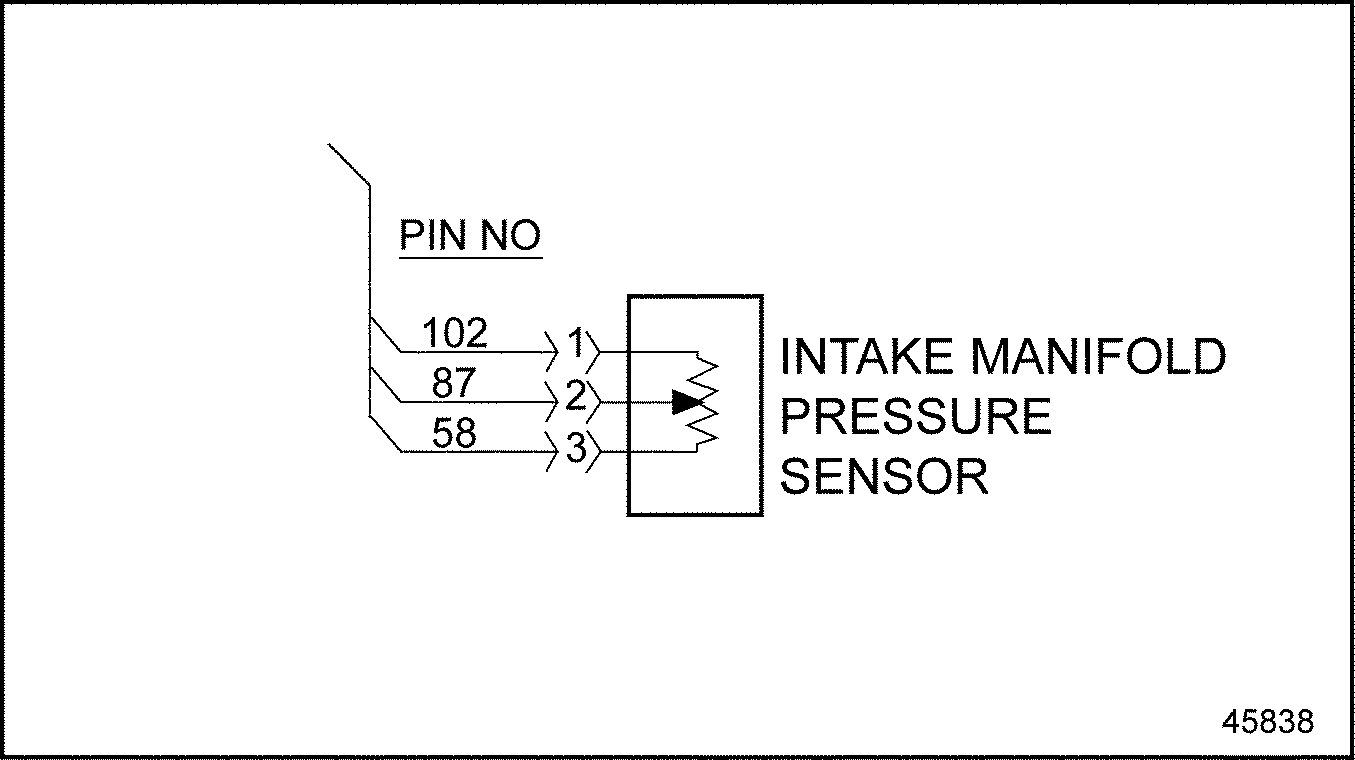# Section 112.4 SPN 3563/FMI 4

### Section 112.4 SPN 3563/FMI 4

The typical diagnosis for this fault is an Intake Manifold Pressure Sensor open circuit.‪

#### Section 112.4.1 Open Circuit Check

Check as follows:‪

1. Disconnect the Intake Manifold Pressure Sensor (IMP Sensor).
2. Measure the resistance between pin 1 and 3 of the IMP Sensor.
1. If resistance is greater than 130 kΩ, replace the IMP Sensor. Refer to "112.4.1.1 Verify Repairs" .
2. If resistance is less than 130 kΩ, go to step 3 .
3. Measure the resistance between pins 1 and 2 of the sensor. See Figure "Intake Manifold Pressure Sensor" .Figure 1. Intake Manifold Pressure Sensor

1. If resistance is greater than 130 kΩ, replace the IMP Sensor. Refer to "112.4.1.1 Verify Repairs" .
2. If resistance is less than 130 kΩ, go to step 4 .
4. Turn the ignition switch to the ON position.
5. Measure the voltage between pins 1 and 3 of the IMP Sensor harness connector.
1. If the voltage is between 4.5 and 5.5 volts, go to step 7 .
2. If the voltage is less than 4.5 volts, go to step 6 .
6. Measure the voltage between pin 3 of the IMP Sensor harness connector and ground.
1. If the voltage is between 4.5 and 5.5 volts, repair the open circuit between pin 1 of the IMP Sensor harness connector and pin 105 of the 120–pin MCM connector. Refer to "112.4.1.1 Verify Repairs" .
2. If the voltage is less than 4.5 volts, repair the open circuit between pin 3 of the IMP Sensor harness connector and pin 82 of the 120–pin MCM connector. Refer to "112.4.1.1 Verify Repairs" .
7. Turn the ignition OFF.
8. Disconnect the 120–pin MCM connector.
9. Measure the resistance between pins 1 and 3 of the IMP Sensor harness connector.
1. If the resistance is greater than 5 Ω, go to step 10 .
2. If the resistance is less than 5 Ω, repair the short between pins 105 and 82 of the 120–pin MCM connector. Refer to "112.4.1.1 Verify Repairs" .
10. Measure the resistance between pins 1 and 2 of the IMP Sensor harness connector.
1. If the resistance is greater than 5 Ω, go to step 11 .
2. If the resistance is less than 5 Ω, repair the short between pins 105 and 54 of the 120–pin MCM connector. Refer to "112.4.1.1 Verify Repairs" .
11. Measure the resistance between pin 3 of the IMP Sensor harness connector and ground.
1. If the resistance is greater than 5 Ω, go to step 12 .
2. If the resistance is less than 5 Ω, repair the short between pin 82 of the 120–pin MCM connector and ground. Refer to "112.4.1.1 Verify Repairs" .
12. Measure the resistance between pin 2 of the IMP Sensor harness connector and ground.
1. If the resistance is greater than 5 Ω, repair the open circuit between pin 2 of the IMP Sensor harness connector and pin 54 of the 120–pin MCM connector. Refer to "112.4.1.1 Verify Repairs" .
2. If the resistance is less than 5 Ω, repair the short between pin 54 of the 120–pin MCM connector. Refer to "112.4.1.1 Verify Repairs" .
##### Section 112.4.1.1 Verify Repairs

Verify repairs as follows:‪

1. Turn ignition OFF.
2. Reconnect any electrical connections that were disconnected to perform the diagnosis.